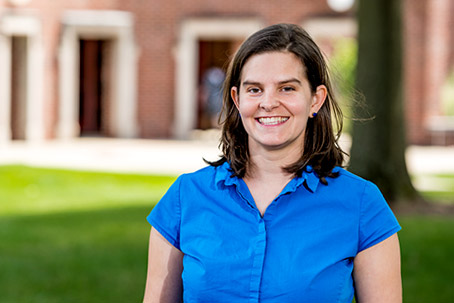# Sylvia CarlisleDr. Sylvia Carlisle's specialties include mathematical logic, model theory for metric structures and continuous logic, and model theory of R-trees. She advises the Alpha Phi Omega service organization and has helped organize the Rose-Hulman Undergraduate Mathematics Conference, which spotlights and celebrates the work of undergraduate mathematicians, and the Alfred R. Schmidt Freshman Mathematics Competition.

## Teaching Interests

• Calculus
• Differential equations
• Discrete mathematics
• Real analysis
• Abstract algebra
• Topology
• Anything to do with proofs

## Research Interests

• Model theory of real trees
• Mathematical logic
• Model theory for metric structures and continuous logic